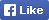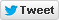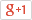Computer/Electronics Questions and Answers
C, C++, VC++, COM/DCOM, DLL and moreLoginSign upHome Electronics Computer Questions Member's Area News Site Map About

Write a program to find the fibonacci numbers upto a given limit using functions

Source Code

 1 #include 2 #include 3 void fibonacci(int r) 4 { 5 int i=0,a=0,b=1; 6 int ar; 7 for(i=0;i<100;i++) 8 { 9 ar[i]=0; 10 } 11 ar=1; 12 for(i=2;i

Find More from our code collection
Armstrong number, binary number to a decimal number, bubble sort, decimal number to binary number, factorial of the given number factors, fibonacci numbers, HCF and LCM, matrix, mergesort, salary of the employee. palindrome, quadratic equation, star patterns, series etc. and much more...Return to Example Source Code